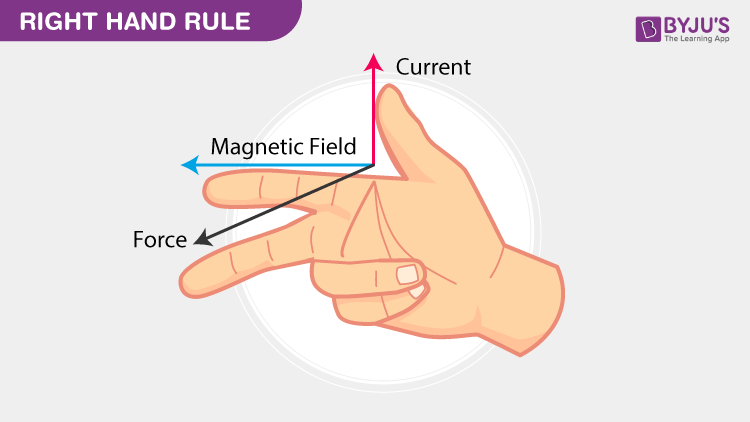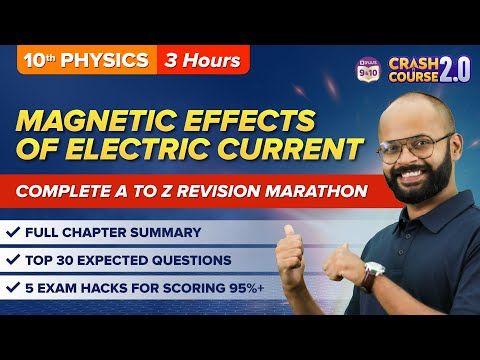# Lorentz Force

Hendrik Antoon Lorentz was a Dutch physicist who explained the theories related to electromagnetic radiation. He mainly concentrated on the relationship between magnetism, light, and electricity.

## What Is Lorentz Force?

Lorentz force is defined as the combination of the magnetic and electric force on a point charge due to electromagnetic fields. It is used in electromagnetism and is also known as electromagnetic force. In the year 1895, Hendrik Lorentz derived the modern formula of Lorentz force.

## Lorentz Force Formula

Lorentz force formula for the charged particle is as follows:

 $$\begin{array}{l}F=q(E+v*B)\end{array}$$

Where,

• F is the force acting on the particle
• q is the electric charge of the particle
• v is the velocity
• E is the external electric field
• B is the magnetic field

Lorentz force formula for continuous charge distribution is as follows:

 $$\begin{array}{l}dF=dq(E+v*B)\end{array}$$

Where,

• dF is a force on a small piece of the charge
• dq is the charge of a small piece

When a small piece of charge distribution is divided by the volume dV, the following is the formula:

 $$\begin{array}{l}f = \rho \,\, (E+v*B)\end{array}$$

Where,

• f is the force per unit volume
• ⍴ is the charge density

With the help of the right-hand rule, it becomes easy to find the direction of the magnetic part of the force.

## Watch the video and learn about the force on a moving charge in a magnetic field### What Is the importance of the Lorentz force?

Lorentz’s force explains the mathematical equations along with the physical importance of forces acting on the charged particles that are travelling through the space containing electric as well as the magnetic field. This is the importance of the Lorentz force.

### Right-Hand Rule

The right-hand rule is useful to find the magnetic force as it becomes easy to visualize the direction as given in Lorentz force law.From the above figure, it is understood that the magnetic force is perpendicular to both the magnetic field and charge velocity.

Related Articles:

## Applications of Lorentz Force

The following are the applications of Lorentz force:

• Cyclotrons and other particle accelerators use Lorentz force.
• A bubble chamber uses Lorentz force to produce the graph for getting the trajectories of charged particles.
• Cathode ray tube televisions use the concept of Lorentz force to deviate the electrons in a straight line so they land on specific spots on the screen.

## Watch the video and learn about cathode ray tube experiment## Frequently Asked Questions – FAQs

Q1

### What is the Lorentz force?

The force experienced on a charged particle due to electric and magnetic fields is known as the Lorentz force.

Q2

F=q(E+v∗B)

Q3

### Lorentz force is named after whom?

The Lorentz force is named after Hendrik Lorentz.

Q4

### 1 tesla is equal to how many gauss?

A Tesla is 10,000 Gauss.
Q5

Right-Hand Rule.

## The below video helps to revise the chapter Magnetic Effects of Electric Current Class 10Stay tuned to BYJU’S and Fall in Love with Learning!

Test your knowledge on Lorentz Force